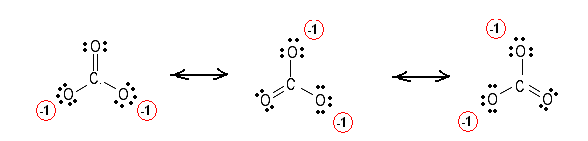Simple Procedure for writing Lewis Structures – Examples #1 | Chemistry Net

# Simple Procedure for writing Lewis Structures – Examples #1

A simple procedure for writing Lewis structures is given in a previous article entitled “Lewis Structures and the Octet Rule”.
Examples for writing Lewis structures following the above procedure are given bellow:

Consider the case of ozone O3. Ozone is a very reactive gas, and even at low concentrations it is irritating and toxic. Event though it represents only a tiny fraction of the atmosphere is crucial for life on earth. Let us draw the Lewis Structures for ozone:

Step1: The central atom will be one of the oxygen atoms.  Connect the 3 atoms with a single bonds

O – O – O

Step 2: Calculate the # of electrons in π bonds (pi bonds, multiple bonds) using formula (1) in the article entitled “Lewis Structures and the Octet Rule”.

Where n in this case is 3 since O3 consists of three atoms
Where V = (6 + 6 + 6 )  = 18
Therefore, P = 6n + 2 – V = 6 * 3 + 2 – 18 = 2      So  there are 2 π electrons in O3  and therefore 1 double bond must be added to the structure of Step 1.

Step 3 & 4:  The 2 atoms are joined together with a double bond. Therefore the Lewis structure for O3 is as follows:

﻿﻿﻿Figure 1: Lewis structures for O3. There are two equivalent resonance structures so in the molecule the bond between the O atoms is something between a double and a single bond. This fact has been proved experimentally.

Consider the case of the carbonate ion, CO3-2

Step1: The central atom will be the C atom since it is the only atom with “subscript” equal to 1 in the molecular formula.  Connect the O atoms with the C atom with single bonds

Step 2: Calculate the # of electrons in π bonds (pi, multiple bonds) using  formula (1):

Where n in this case is 4 since CO3-2 consists of four atoms
Where V = (4 + 6 + 6 + 6 ) – (-2)  = 24
Therefore, P = 6n + 2 – V = 6 * 4 + 2 – 24 = 2      \  there are 2 π electrons (pi electrons) in  CO3-2  and therefore 1 double   bond must be added to the structure of Step 1.

Step 3 & 4: One double bond between C and O is added to the structure in step 1. Unshared electron pairs are added so that there is an octet of electrons around each atom. All the equivalent resonance structures are drawn by delocalizing electron pairs. Therefore, the Lewis structures for CO3-2  are as follows:Figure 2: Lewis structures for the carbonate ion CO3-2. The 3 structures are equivalent since they have equal formal charges on the elements. It has been proven experimentally that the C-O bond in the carbonate ion is a hybrid of a single and a double bond (the length of the C-O bond in the carbonate ion is approximately half of the sum of the lengths of a normal C-O and C=O bond).This means that the theoretical Lewis structures are in agreement with experimental results.